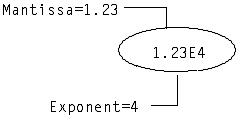Floating Point Data Types
A floating point value is represented either as whole plus fractional digits (like decimal values) or as a mantissa plus an exponent.
The following is an example of the mantissa and exponent parts of floating point values:There are two floating point data types:
float4 (4-byte)
float (8-byte)
A synonym for float4 is real. Synonyms for float are float8 and double precision.
Floating point numbers are stored in four or eight bytes. Internally, eight-byte numbers are rounded to fifteen decimal digits. The precision of four-byte numbers is processor dependent.
You can specify the minimum required binary precision (number of significant bits) for a floating point value using the following optional syntax:
float(n)
where n is a value from 0 to 53. Storage is allocated based on the precision that is specified, as follows:
Range of Binary Precision
Storage Allocated
Example
0 to 23
4-byte float
float(18) defines a floating point type with at least 18 binary digits of precision in the mantissa. A 4‑byte floating point field is allocated for it, which has 23 bits of precision.
24 to 53
8-byte float
float(41) defines a floating point type with at least 41 binary digits of precision in the mantissa. A 8‑byte floating point field is allocated for it, which has 53 bits of precision.
Floating point precision is not limited to the declared size.
Float Point Limitations
You must consider the effects of data type conversions when numeric values are combined or compared. This is especially true when dealing with floating point values.
Exact matches on floating point numbers are discouraged, because float and float4 data types are approximate numeric values. In contrast, integer and decimal data types are exact numeric values.
Here is an example of why it is hard to find an exact match on a floating point number:
CREATE TABLE test(f8 FLOAT8);
INSERT INTO test VALUES(330/11.0);
SELECT * FROM test WHERE f8 = 30;
returns no rows.
On inspection,
SELECT HEX(f8) FROM test;
returns:
+----------------+
|col1            |
+----------------+
|403E000000000001|
+----------------+
Using an IEEE analyzer we find this number to be decimal 30.000000000000003552713678800500929355621337890625, which, though close to 30, shows why no exact match was found.
To ensure the query returns a row, you can change it to the following:
SELECT * FROM test WHERE ABS(f8 - 30) < 0.0000000001